# Rectangles

How many different rectangles with sides integers (in mm) have a circumference exactly 1000 cm?

Result

n =  2500

#### Solution:Leave us a comment of example and its solution (i.e. if it is still somewhat unclear...):

Showing 0 comments:Be the first to comment!#### To solve this example are needed these knowledge from mathematics:

Do you want to convert length units?

## Next similar examples:

1. Tablecloth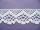The table top is 90 cm long and 50 cm wide. How many cm lace edging is necessary for the tablecloth that hangs on each side by 10 cm?
2. The door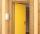The door to the apartment has a height 2 m and a width 80 cm. Calculate how many cm of sealing tape is needed to seal them. Will there be enough packaging in which there are 5 m tape (write 0 = no, 1 = yes)?
3. Fence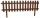How many meters of fencing it is required to purchase if our garden is rectangular measuring 20 m and 180 dm.
4. Foot in busIt was 102 people on the bus. 28 girls had two dogs. A 11 girls had one dog. At the next stop seceded 5 dogs (even with their owners). They got two boys together with three dogs. The bus drove one driver. How many foot were in bus?
5. Bean bag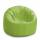A student tossed a bean bag. It landed 216 inches away. How many yards are equal to 216 inches?
6. Class alphabet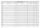All 29 pupils in the class are written in a class by alphabet. The number of pupils enrolled before Paul is three times higher than the number of pupils behind him. Calculate how many pupils are enrolled after Paul.
7. Wood bars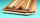It is 9 bars 14 meters long and 9 bars 11 meters long. How many 5-meter lath is needed to make them?
8. RectangleCalculate perimeter of the rectangle with sides a=2.4 m and b=1.9 m.
9. PlaygroundRectangular playground is fenced with 38 m long netting. Its width is 7 m. Calculate its length.
10. Table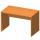Find the circumference of the table, where the long side is 1.28 meters and the short side is 86 cm.
11. Zahrada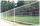Zahrada má rozměry 5m a 400cm. Kolik pletiva třeba na oplocení pozemku?
12. RectangleThe rectangle area is 182 dm2, its base is 14 dm. How long is the other side? Calculate its perimeter.
13. DiamondThe diamond has a circumference of 48cm. Calculate the length of its side in dm.
14. Addition of Roman numbersAdded together and write as decimal number: LXVII + MLXIV
15. CagesHonza had three cages (black, silver, gold) and three animals (guinea pig, rat and puppy). There was one animal in each cage. The golden cage stood to the left of the black cage. The silver cage stood on the right of the guinea pig cage. The rat was in the
16. Wire cut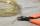A wire of length 7 m was cut into equal lengths using 4 cuts. How long is each piece?
17. RhombusFind the length of each side of rhombus if the perimeter is 49 dm long.